# bezier.triangle module¶

Helper for Bézier Triangles.

class bezier.triangle.Triangle(nodes, degree, *, copy=True, verify=True)

Bases: bezier._base.Base

Represents a Bézier triangle.

We define a Bézier triangle as a mapping from the unit simplex in $$\mathbf{R}^2$$ (i.e. the unit triangle) onto a triangle in an arbitrary dimension. We use barycentric coordinates

$\lambda_1 = 1 - s - t, \lambda_2 = s, \lambda_3 = t$

for points in the unit triangle $$\left\{(s, t) \mid 0 \leq s, t, s + t \leq 1\right\}$$: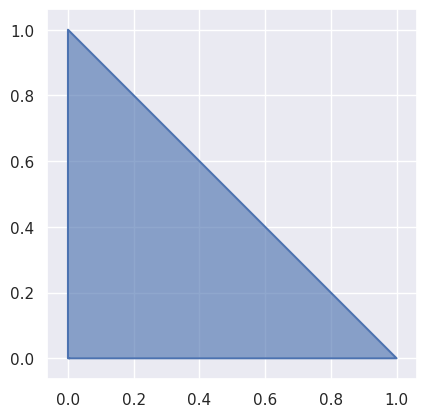As with curves, using these weights we get convex combinations of points $$v_{i, j, k}$$ in some vector space:

$B\left(\lambda_1, \lambda_2, \lambda_3\right) = \sum_{i + j + k = d} \binom{d}{i \, j \, k} \lambda_1^i \lambda_2^j \lambda_3^k \cdot v_{i, j, k}$

Note

We assume the nodes are ordered from left-to-right and from bottom-to-top. So for example, the linear triangle:

(0,0,1)

(1,0,0)  (0,1,0)


is ordered as

$\left[\begin{array}{c c c} v_{1,0,0} & v_{0,1,0} & v_{0,0,1} \end{array}\right]$

(0,0,2)

(1,0,1)  (0,1,1)

(2,0,0)  (1,1,0)  (0,2,0)


is ordered as

$\left[\begin{array}{c c c c c c} v_{2,0,0} & v_{1,1,0} & v_{0,2,0} & v_{1,0,1} & v_{0,1,1} & v_{0,0,2} \end{array}\right]$

the cubic triangle:

(0,0,3)

(1,0,2)  (0,1,2)

(2,0,1)  (1,1,1)  (0,2,1)

(3,0,0)  (2,1,0)  (1,2,0)  (0,3,0)


is ordered as

$\left[\begin{array}{c c c c c c c c c c} v_{3,0,0} & v_{2,1,0} & v_{1,2,0} & v_{0,3,0} & v_{2,0,1} & v_{1,1,1} & v_{0,2,1} & v_{1,0,2} & v_{0,1,2} & v_{0,0,3} \end{array}\right]$

and so on.

The index formula

$j + \frac{k}{2} \left(2 (i + j) + k + 3\right)$

can be used to map a triple $$(i, j, k)$$ onto the corresponding linear index, but it is not particularly insightful or useful.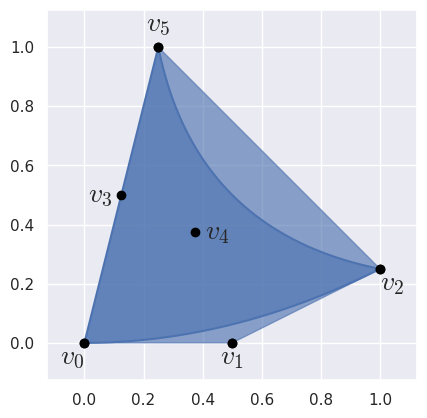>>> import bezier
>>> nodes = np.asfortranarray([
...     [0.0, 0.5, 1.0 , 0.125, 0.375, 0.25],
...     [0.0, 0.0, 0.25, 0.5  , 0.375, 1.0 ],
... ])
>>> triangle = bezier.Triangle(nodes, degree=2)
>>> triangle
<Triangle (degree=2, dimension=2)>

Parameters
• nodes (Sequence [ Sequence [ numbers.Number ] ]) – The nodes in the triangle. Must be convertible to a 2D NumPy array of floating point values, where the columns represent each node while the rows are the dimension of the ambient space.

• degree (int) – The degree of the triangle. This is assumed to correctly correspond to the number of nodes. Use from_nodes() if the degree has not yet been computed.

• copy (bool) – Flag indicating if the nodes should be copied before being stored. Defaults to True since callers may freely mutate nodes after passing in.

• verify (bool) – Flag indicating if the degree should be verified against the number of nodes. Defaults to True.

classmethod from_nodes(nodes, copy=True)

Create a Triangle from nodes.

Computes the degree based on the shape of nodes.

Parameters
Returns

The constructed triangle.

Return type

Triangle

property area

The area of the current triangle.

For triangles in $$\mathbf{R}^2$$, this computes the area via Green’s theorem. Using the vector field $$\mathbf{F} = \left[-y, x\right]^T$$, since $$\partial_x(x) - \partial_y(-y) = 2$$ Green’s theorem says twice the area is equal to

$\int_{B\left(\mathcal{U}\right)} 2 \, d\mathbf{x} = \int_{\partial B\left(\mathcal{U}\right)} -y \, dx + x \, dy.$

This relies on the assumption that the current triangle is valid, which implies that the image of the unit triangle under the Bézier map — $$B\left(\mathcal{U}\right)$$ — has the edges of the triangle as its boundary.

Note that for a given edge $$C(r)$$ with control points $$x_j, y_j$$, the integral can be simplified:

$\int_C -y \, dx + x \, dy = \int_0^1 (x y' - y x') \, dr = \sum_{i < j} (x_i y_j - y_i x_j) \int_0^1 b_{i, d} b'_{j, d} \, dr$

where $$b_{i, d}, b_{j, d}$$ are Bernstein basis polynomials.

Returns

The area of the current triangle.

Return type

float

Raises

NotImplementedError – If the current triangle isn’t in $$\mathbf{R}^2$$.

property edges

The edges of the triangle.

>>> nodes = np.asfortranarray([
...     [0.0,  0.5   , 1.0, 0.1875, 0.625, 0.0],
...     [0.0, -0.1875, 0.0, 0.5   , 0.625, 1.0],
... ])
>>> triangle = bezier.Triangle(nodes, degree=2)
>>> edge1, _, _ = triangle.edges
>>> edge1
<Curve (degree=2, dimension=2)>
>>> edge1.nodes
array([[ 0. ,  0.5   , 1. ],
[ 0. , -0.1875, 0. ]])

Returns

The edges of the triangle.

Return type
evaluate_barycentric(lambda1, lambda2, lambda3, _verify=True)

Compute a point on the triangle.

Evaluates $$B\left(\lambda_1, \lambda_2, \lambda_3\right)$$.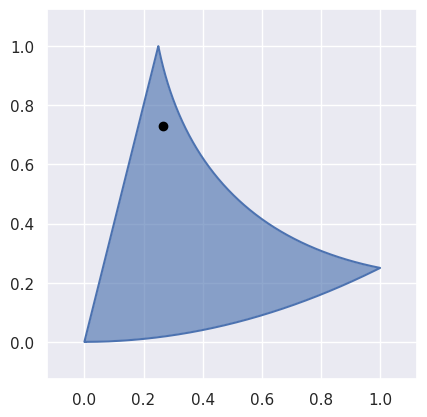>>> nodes = np.asfortranarray([
...     [0.0, 0.5, 1.0 , 0.125, 0.375, 0.25],
...     [0.0, 0.0, 0.25, 0.5  , 0.375, 1.0 ],
... ])
>>> triangle = bezier.Triangle(nodes, degree=2)
>>> point = triangle.evaluate_barycentric(0.125, 0.125, 0.75)
>>> point
array([[0.265625  ],
[0.73046875]])


However, this can’t be used for points outside the reference triangle:

>>> triangle.evaluate_barycentric(-0.25, 0.75, 0.5)
Traceback (most recent call last):
...
ValueError: ('Weights must be positive', -0.25, 0.75, 0.5)


or for non-barycentric coordinates;

>>> triangle.evaluate_barycentric(0.25, 0.25, 0.25)
Traceback (most recent call last):
...
ValueError: ('Weights do not sum to 1', 0.25, 0.25, 0.25)


However, these “invalid” inputs can be used if _verify is False.

>>> triangle.evaluate_barycentric(-0.25, 0.75, 0.5, _verify=False)
array([[0.6875  ],
[0.546875]])
>>> triangle.evaluate_barycentric(0.25, 0.25, 0.25, _verify=False)
array([[0.203125],
[0.1875  ]])

Parameters
• lambda1 (float) – Parameter along the reference triangle.

• lambda2 (float) – Parameter along the reference triangle.

• lambda3 (float) – Parameter along the reference triangle.

• _verify (Optional [ bool ]) – Indicates if the barycentric coordinates should be verified as summing to one and all non-negative (i.e. verified as barycentric). Can either be used to evaluate at points outside the domain, or to save time when the caller already knows the input is verified. Defaults to True.

Returns

The point on the triangle (as a two dimensional NumPy array with a single column).

Return type

numpy.ndarray

Raises
• ValueError – If the weights are not valid barycentric coordinates, i.e. they don’t sum to 1. (Won’t raise if _verify=False.)

• ValueError – If some weights are negative. (Won’t raise if _verify=False.)

evaluate_barycentric_multi(param_vals, _verify=True)

Compute multiple points on the triangle.

Assumes param_vals has three columns of barycentric coordinates. See evaluate_barycentric() for more details on how each row of parameter values is evaluated.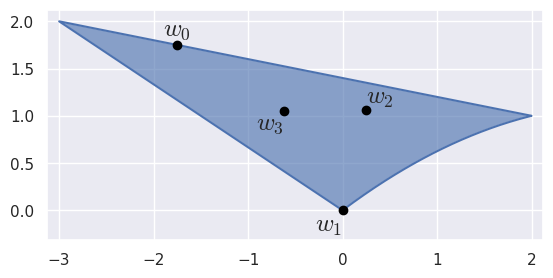>>> nodes = np.asfortranarray([
...     [0.0, 1.0 , 2.0, -1.5, -0.5, -3.0],
...     [0.0, 0.75, 1.0,  1.0,  1.5,  2.0],
... ])
>>> triangle = bezier.Triangle(nodes, degree=2)
>>> triangle
<Triangle (degree=2, dimension=2)>
>>> param_vals = np.asfortranarray([
...     [0.   , 0.25, 0.75 ],
...     [1.   , 0.  , 0.   ],
...     [0.25 , 0.5 , 0.25 ],
...     [0.375, 0.25, 0.375],
... ])
>>> points = triangle.evaluate_barycentric_multi(param_vals)
>>> points
array([[-1.75 , 0. , 0.25   , -0.625   ],
[ 1.75 , 0. , 1.0625 ,  1.046875]])

Parameters
Returns

The points on the triangle.

Return type

numpy.ndarray

Raises

ValueError – If param_vals is not a 2D array and _verify=True.

evaluate_cartesian(s, t, _verify=True)

Compute a point on the triangle.

Evaluates $$B\left(1 - s - t, s, t\right)$$ by calling evaluate_barycentric():

This method acts as a (partial) inverse to locate().

>>> nodes = np.asfortranarray([
...     [0.0, 0.5, 1.0  , 0.0, 0.5, 0.25],
...     [0.0, 0.5, 0.625, 0.5, 0.5, 1.0 ],
... ])
>>> triangle = bezier.Triangle(nodes, degree=2)
>>> point = triangle.evaluate_cartesian(0.125, 0.375)
>>> point
array([[0.16015625],
[0.44726562]])
>>> triangle.evaluate_barycentric(0.5, 0.125, 0.375)
array([[0.16015625],
[0.44726562]])

Parameters
Returns

The point on the triangle (as a two dimensional NumPy array).

Return type

numpy.ndarray

evaluate_cartesian_multi(param_vals, _verify=True)

Compute multiple points on the triangle.

Assumes param_vals has two columns of Cartesian coordinates. See evaluate_cartesian() for more details on how each row of parameter values is evaluated.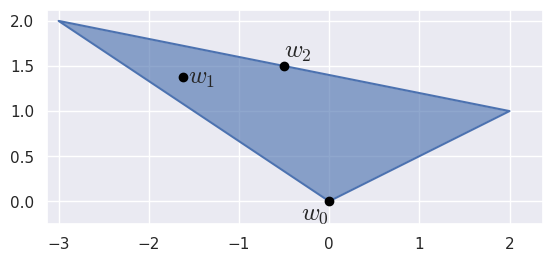>>> nodes = np.asfortranarray([
...     [0.0, 2.0, -3.0],
...     [0.0, 1.0,  2.0],
... ])
>>> triangle = bezier.Triangle(nodes, degree=1)
>>> triangle
<Triangle (degree=1, dimension=2)>
>>> param_vals = np.asfortranarray([
...     [0.0  , 0.0  ],
...     [0.125, 0.625],
...     [0.5  , 0.5  ],
... ])
>>> points = triangle.evaluate_cartesian_multi(param_vals)
>>> points
array([[ 0. , -1.625, -0.5 ],
[ 0. ,  1.375,  1.5 ]])

Parameters
Returns

The points on the triangle.

Return type

numpy.ndarray

Raises

ValueError – If param_vals is not a 2D array and _verify=True.

plot(pts_per_edge, color=None, ax=None, with_nodes=False)

Plot the current triangle.

Parameters
Returns

The axis containing the plot. This may be a newly created axis.

Return type

matplotlib.artist.Artist

Raises

NotImplementedError – If the triangle’s dimension is not 2.

subdivide()

Split the triangle into four sub-triangles.

Does so by taking the unit triangle (i.e. the domain of the triangle) and splitting it into four sub-triangles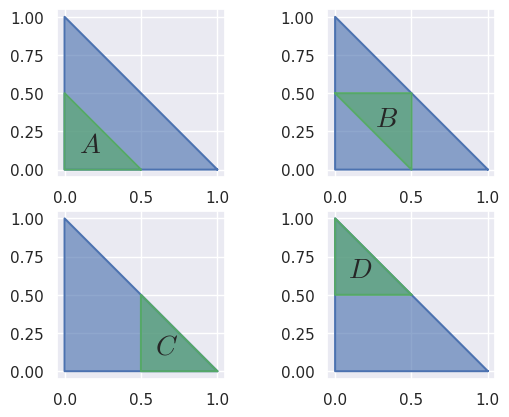Then the triangle is re-parameterized via the map to / from the given sub-triangles and the unit triangle.

For example, when a degree two triangle is subdivided: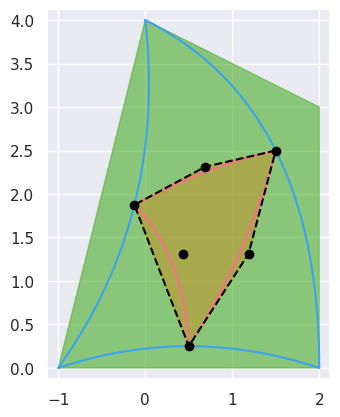>>> nodes = np.asfortranarray([
...     [-1.0, 0.5, 2.0, 0.25, 2.0, 0.0],
...     [ 0.0, 0.5, 0.0, 1.75, 3.0, 4.0],
... ])
>>> triangle = bezier.Triangle(nodes, degree=2)
>>> _, sub_triangle_b, _, _ = triangle.subdivide()
>>> sub_triangle_b
<Triangle (degree=2, dimension=2)>
>>> sub_triangle_b.nodes
array([[ 1.5 ,  0.6875, -0.125 , 1.1875, 0.4375, 0.5  ],
[ 2.5 ,  2.3125,  1.875 , 1.3125, 1.3125, 0.25 ]])

Returns

The lower left, central, lower right and upper left sub-triangles (in that order).

Return type
property is_valid

Flag indicating if the triangle is “valid”.

Here, “valid” means there are no self-intersections or singularities and the edges are oriented with the interior (i.e. a 90 degree rotation of the tangent vector to the left is the interior).

This checks if the Jacobian of the map from the reference triangle is everywhere positive. For example, a linear “triangle” with collinear points is invalid: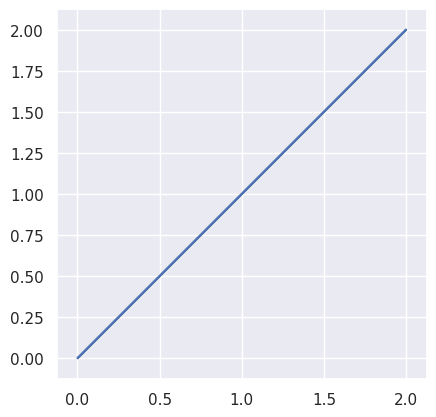>>> nodes = np.asfortranarray([
...     [0.0, 1.0, 2.0],
...     [0.0, 1.0, 2.0],
... ])
>>> triangle = bezier.Triangle(nodes, degree=1)
>>> triangle.is_valid
False


while a quadratic triangle with one straight side: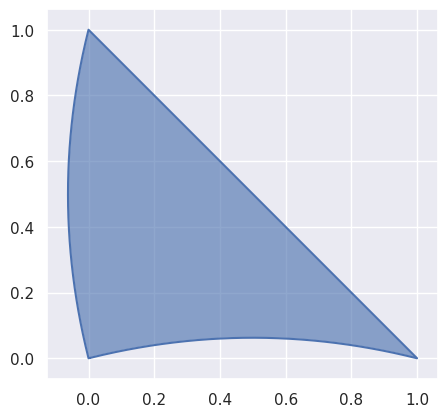>>> nodes = np.asfortranarray([
...     [0.0, 0.5  , 1.0, -0.125, 0.5, 0.0],
...     [0.0, 0.125, 0.0,  0.5  , 0.5, 1.0],
... ])
>>> triangle = bezier.Triangle(nodes, degree=2)
>>> triangle.is_valid
True


though not all higher degree triangles are valid: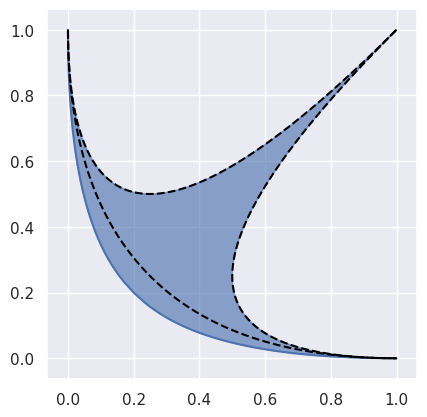>>> nodes = np.asfortranarray([
...     [1.0, 0.0, 1.0, 0.0, 0.0, 0.0],
...     [0.0, 0.0, 1.0, 0.0, 0.0, 1.0],
... ])
>>> triangle = bezier.Triangle(nodes, degree=2)
>>> triangle.is_valid
False

Type

bool

locate(point, _verify=True)

Find a point on the current triangle.

Solves for $$s$$ and $$t$$ in $$B(s, t) = p$$.

This method acts as a (partial) inverse to evaluate_cartesian().

Warning

A unique solution is only guaranteed if the current triangle is valid. This code assumes a valid triangle, but doesn’t check.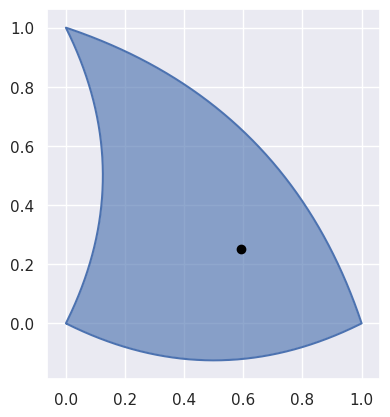>>> nodes = np.asfortranarray([
...     [0.0,  0.5 , 1.0, 0.25, 0.75, 0.0],
...     [0.0, -0.25, 0.0, 0.5 , 0.75, 1.0],
... ])
>>> triangle = bezier.Triangle(nodes, degree=2)
>>> point = np.asfortranarray([
...     [0.59375],
...     [0.25   ],
... ])
>>> s, t = triangle.locate(point)
>>> s
0.5
>>> t
0.25

Parameters
Returns

The $$s$$ and $$t$$ values corresponding to point or None if the point is not on the triangle.

Return type
Raises
• NotImplementedError – If the triangle isn’t in $$\mathbf{R}^2$$.

• ValueError – If the dimension of the point doesn’t match the dimension of the current triangle.

intersect(other, strategy=<IntersectionStrategy.GEOMETRIC: 0>, _verify=True)

Find the common intersection with another triangle.

Parameters
Returns

List of intersections (possibly empty).

Return type
Raises
elevate()

Return a degree-elevated version of the current triangle.

Does this by converting the current nodes $$\left\{v_{i, j, k}\right\}_{i + j + k = d}$$ to new nodes $$\left\{w_{i, j, k}\right\}_{i + j + k = d + 1}$$. Does so by re-writing

$E\left(\lambda_1, \lambda_2, \lambda_3\right) = \left(\lambda_1 + \lambda_2 + \lambda_3\right) B\left(\lambda_1, \lambda_2, \lambda_3\right) = \sum_{i + j + k = d + 1} \binom{d + 1}{i \, j \, k} \lambda_1^i \lambda_2^j \lambda_3^k \cdot w_{i, j, k}$

In this form, we must have

\begin{split}\begin{align*} \binom{d + 1}{i \, j \, k} \cdot w_{i, j, k} &= \binom{d}{i - 1 \, j \, k} \cdot v_{i - 1, j, k} + \binom{d}{i \, j - 1 \, k} \cdot v_{i, j - 1, k} + \binom{d}{i \, j \, k - 1} \cdot v_{i, j, k - 1} \\ \Longleftrightarrow (d + 1) \cdot w_{i, j, k} &= i \cdot v_{i - 1, j, k} + j \cdot v_{i, j - 1, k} + k \cdot v_{i, j, k - 1} \end{align*}\end{split}

where we define, for example, $$v_{i, j, k - 1} = 0$$ if $$k = 0$$.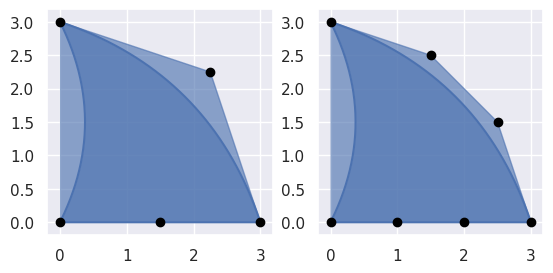>>> nodes = np.asfortranarray([
...     [0.0, 1.5, 3.0, 0.75, 2.25, 0.0],
...     [0.0, 0.0, 0.0, 1.5 , 2.25, 3.0],
... ])
>>> triangle = bezier.Triangle(nodes, degree=2)
>>> elevated = triangle.elevate()
>>> elevated
<Triangle (degree=3, dimension=2)>
>>> elevated.nodes
array([[0. , 1. , 2. , 3. , 0.5 , 1.5 , 2.5 , 0.5 , 1.5 , 0. ],
[0. , 0. , 0. , 0. , 1.  , 1.25, 1.5 , 2.  , 2.5 , 3. ]])

Returns

The degree-elevated triangle.

Return type

Triangle

to_symbolic()

Convert to a SymPy matrix representing $$B(s, t)$$.

Note

This method requires SymPy.

>>> nodes = np.asfortranarray([
...     [0.0, 0.5, 1.0, -0.5, 0.0, -1.0],
...     [0.0, 0.0, 1.0,  0.0, 0.0,  0.0],
...     [0.0, 0.0, 0.0,  0.0, 0.0,  1.0],
... ])
>>> triangle = bezier.Triangle(nodes, degree=2)
>>> triangle.to_symbolic()
Matrix([
[s - t],
[ s**2],
[ t**2]])

Returns

The triangle $$B(s, t)$$.

Return type

sympy.Matrix

implicitize()

Implicitize the triangle .

Note

This method requires SymPy.

>>> nodes = np.asfortranarray([
...     [0.0, 0.5, 1.0, -0.5, 0.0, -1.0],
...     [0.0, 0.0, 1.0,  0.0, 0.0,  0.0],
...     [0.0, 0.0, 0.0,  0.0, 0.0,  1.0],
... ])
>>> triangle = bezier.Triangle(nodes, degree=2)
>>> triangle.implicitize()
(x**4 - 2*x**2*y - 2*x**2*z + y**2 - 2*y*z + z**2)**2

Returns

The function that defines the triangle in $$\mathbf{R}^3$$ via $$f(x, y, z) = 0$$.

Return type

sympy.Expr

Raises

ValueError – If the triangle’s dimension is not 3.

property degree

The degree of the current shape.

Type

int

property dimension

The dimension that the shape lives in.

For example, if the shape lives in $$\mathbf{R}^3$$, then the dimension is 3.

Type

int

property nodes

The nodes that define the current shape.

Type

numpy.ndarray